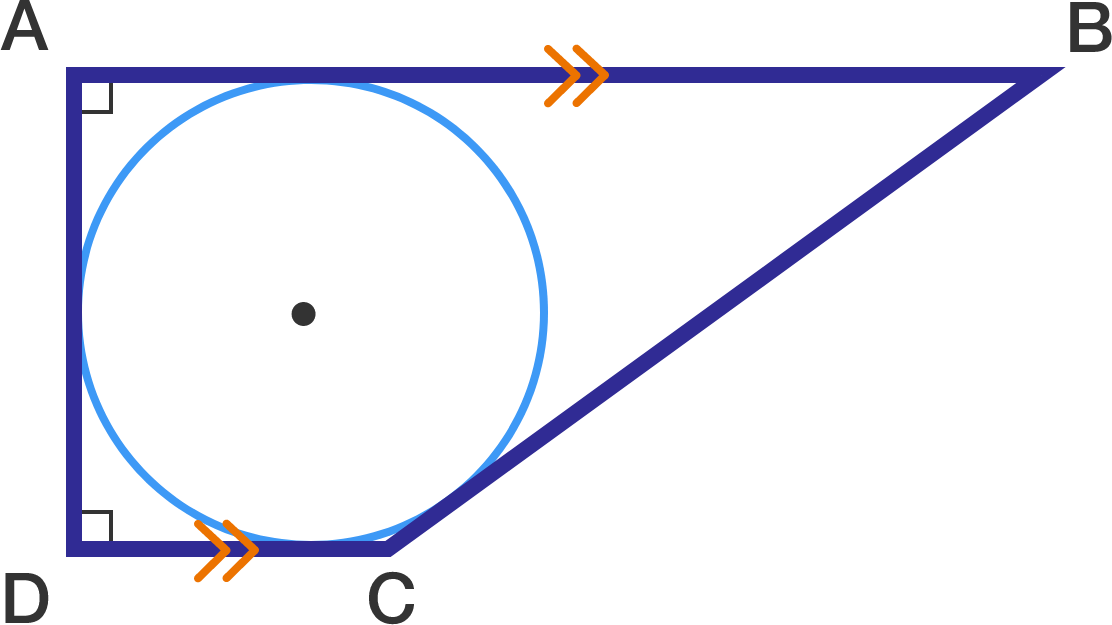# Circle in a trapezium

Geometry Level 4A circle is inscribed inside a trapezium $ABCD$ such that it touches all 4 sides of this trapezium, as shown above.

Given that the area of this trapezium is 4 and the diameter of the circle is 1, find the value of the ratio $\dfrac {AB}{CD}$.

Give your answer to 2 decimal places.

Clarification: $AB$ is longer than $CD$.

×

Problem Loading...

Note Loading...

Set Loading...